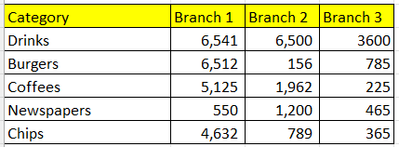cancel
Showing results for
Did you mean:

Fabric is Generally Available. Browse Fabric Presentations. Work towards your Fabric certification with the Cloud Skills Challenge.Regular Visitor

## How to find difference of two identical tables in Power BI Visualisation Matrix

Hi Guys, I am new to power BI. I got visualization of two tables using 'Matrix' option in build visual.

1. Actual Sales

2. Target Sales

Actual SalesTarget SalesNow, I'm struggling to create another 'Matrix' visual table that can give me difference of two tables.

3. Difference in table values.I can't find any visuals, that could help be do this subtraction between the visuals tables ?Cheers!

1 ACCEPTED SOLUTIONCommunity Support

Hi @vivek09 ,

1. create measure with below dax formula

``````Branch1 =
VAR cur_cty =
SELECTEDVALUE ( 'Target Sales'[Category] )
VAR _a =
CALCULATE (
MAX ( 'Actual Sales'[Branch 1] ),
'Actual Sales'[Category] = cur_cty
)
VAR _b =
CALCULATE (
MAX ( 'Target Sales'[Branch 1] ),
'Target Sales'[Category] = cur_cty
)
RETURN
_a - _b
``````
``````Branch2 =
VAR cur_cty =
SELECTEDVALUE ( 'Target Sales'[Category] )
VAR _a =
CALCULATE (
MAX ( 'Actual Sales'[Branch 2] ),
'Actual Sales'[Category] = cur_cty
)
VAR _b =
CALCULATE (
MAX ( 'Target Sales'[Branch 2] ),
'Target Sales'[Category] = cur_cty
)
RETURN
_a - _b
``````
``````Branch3 =
VAR cur_cty =
SELECTEDVALUE ( 'Target Sales'[Category] )
VAR _a =
CALCULATE (
MAX ( 'Actual Sales'[Branch 3] ),
'Actual Sales'[Category] = cur_cty
)
VAR _b =
CALCULATE (
MAX ( 'Target Sales'[Branch 3] ),
'Target Sales'[Category] = cur_cty
)
RETURN
_a - _b
``````
``````A =
VAR _a = [Branch1] RETURN IF ( _a > 0, "black", "red" )
``````
``````B =
VAR _a = [Branch2] RETURN IF ( _a > 0, "black", "red" )
``````
``````C =
VAR _a = [Branch3] RETURN IF ( _a > 0, "black", "red" )
``````

2. add a matrix visual with table filed and measure, apply A,B,C measure to conditional format for font colorPlease refer the attached .pbix file.

Best regards,
Community Support Team_Binbin Yu
If this post helps, then please consider Accept it as the solution to help the other members find it more quickly.

4 REPLIES 4Community Support

Hi @vivek09 ,

1. create measure with below dax formula

``````Branch1 =
VAR cur_cty =
SELECTEDVALUE ( 'Target Sales'[Category] )
VAR _a =
CALCULATE (
MAX ( 'Actual Sales'[Branch 1] ),
'Actual Sales'[Category] = cur_cty
)
VAR _b =
CALCULATE (
MAX ( 'Target Sales'[Branch 1] ),
'Target Sales'[Category] = cur_cty
)
RETURN
_a - _b
``````
``````Branch2 =
VAR cur_cty =
SELECTEDVALUE ( 'Target Sales'[Category] )
VAR _a =
CALCULATE (
MAX ( 'Actual Sales'[Branch 2] ),
'Actual Sales'[Category] = cur_cty
)
VAR _b =
CALCULATE (
MAX ( 'Target Sales'[Branch 2] ),
'Target Sales'[Category] = cur_cty
)
RETURN
_a - _b
``````
``````Branch3 =
VAR cur_cty =
SELECTEDVALUE ( 'Target Sales'[Category] )
VAR _a =
CALCULATE (
MAX ( 'Actual Sales'[Branch 3] ),
'Actual Sales'[Category] = cur_cty
)
VAR _b =
CALCULATE (
MAX ( 'Target Sales'[Branch 3] ),
'Target Sales'[Category] = cur_cty
)
RETURN
_a - _b
``````
``````A =
VAR _a = [Branch1] RETURN IF ( _a > 0, "black", "red" )
``````
``````B =
VAR _a = [Branch2] RETURN IF ( _a > 0, "black", "red" )
``````
``````C =
VAR _a = [Branch3] RETURN IF ( _a > 0, "black", "red" )
``````

2. add a matrix visual with table filed and measure, apply A,B,C measure to conditional format for font colorPlease refer the attached .pbix file.

Best regards,
Community Support Team_Binbin Yu
If this post helps, then please consider Accept it as the solution to help the other members find it more quickly.Regular Visitor

Thanks very much for this. If I have like 15 to 20 branches, do I need to create individual measures to capture these ? or is there a simpler way for aggregation?

Thank youSuper User

Hi @vivek09 organize your data so in new column you have Branch 1/2/3 so you can slices / show it in column with matrix visual. Hope this help.

Proud to be a Super User!Super User

Hi @vivek09 organize your data so in new column you have Branch 1/2/3 so you can slices / show it in column with matrix visual. Hope this help.

Proud to be a Super User!Announcements#### Power BI Monthly Update - November 2023

Check out the November 2023 Power BI update to learn about new features.#### Fabric Community News unified experience

Read the latest Fabric Community announcements, including updates on Power BI, Synapse, Data Factory and Data Activator.#### The largest Power BI and Fabric virtual conference

130+ sessions, 130+ speakers, Product managers, MVPs, and experts. All about Power BI and Fabric. Attend online or watch the recordings.Top Solution Authors
Top Kudoed Authors
Users online (3,636)